TL;DR: How to (not) get rich? Running Universal Portfolios online with Online Convex Optimization techniques.

The following does not constitute any investment advice.

In 1956 Kelly Jr., at Bell Labs then, wrote a very groundbreaking paper [K] that provided a strong link between Shannon’s (then) newly proposed information theory [S] and gambling (Latane [L] came to similar conclusions around the same time, however published it later). Without going too much into detail here—the casual reader is referred to Poundstone’s popular science account [P] and the more technically inclided reader to [K] and [L]; also make sure to check out [C]—what Kelly showed in the context of sequential betting (his example was horse races) the growth of the bettor’s bankroll is upper bounded by $2^r$, where $r$ is the information rate of the private wire of the bettor, i.e., information that only the bettor is privy to, the information advantage. Moreover, Kelly also proposed an optimal strategy, nowadays called Kelly Strategy or Kelly Betting, that achieves this growth rate and, moreover, for any substantially different strategy, the limit of that strategy’s return divided by the return of the Kelly strategy goes to $0$.

### The Kelly Criterion

Given sequential betting opportunities, e.g., in a casino, the key question is how much money of the current bankroll $V$ to invest. What Kelly showed is that the optimal fraction $f^\esx$ to wager is given by:

$\tag{KellyFraction} f^\esx \doteq \frac{bp - q}{b},$

where $b$ is the net odds (you get $b$ dollars back for each invested dollar on top of the dollar returned to you), $p$ is the probability of winning and $q=1-p$ is the probability of losing. From this formula we can immediately see that we wager a fraction of $0$ if and only if $bp = 1 - p$ or equivalently $p = \frac{1}{b+1}$, i.e., our probability of winning is net flat against the odds, i.e., we have no informational advantage.

However, rather than dwelling on the interpretation as well as the optimality of the above, it is instructive to understand where this formula originates from. In fact, the Kelly fraction naturally arises as the fraction that maximizes the geometric growth of our bankroll under sequential betting or equivalently maximizes the expectation of the logarithm of our (terminal) wealth. The rationelle behind this objective is as follows. Suppose we have an initial backroll of $X_0$ and we sequentially bet at each time step $t$ a given fraction $f$ of our bankroll $X_t$. Let us make the simplifying assumption that the we have even wager bets, i.e., if we win we get our money $f X_t$ back and another $f X_t$ as win and if we lose we lose $f X_t$ as such our bankroll $X_t$ evolves as

$X_n = X_0 (1+f)^S (1-f)^F,$

where $S$ is the number of successes and $F$ the number of failures, i.e., in particular $S + F = n$. From this we obtain a average growth (per bet) of

$\left( \frac{X_n}{X_0} \right)^{1/n} = (1+f)^{S/n} (1-f)^{F/n},$

where $S/n \rightarrow p$ the probability of success and $F/n \rightarrow 1-p$ the probability of failure. The term on the left is the growth rate per bet on average and we want to find an $f$ that maximizes this quantity via the right-hand side. In log-world this is equivalent to

$\frac{1}{n} (\log X_n - \log X_0) = \frac{S}{n} \log (1 + f) + \frac{F}{n} \log (1-f),$

or in the limit for $n$ large we obtain:

$\tag{maxExpLog} \mathbb E[g(f)] = p \log (1 + f) + (1-p) \log (1-f),$

where $g$ is the expected growth rate per bet when betting fraction $f$, which is independent of $n$ now. Note, that it can be also easily seen that betting a fixed fraction $f$ (i.e., independent of time) here is sufficient provided that the individual bets are independent and we care for the (maximization of the) expected growth rate.

Now we can simply maximize the right-hand side by computing a critical point:

$0 = p \frac{1}{1+f} - (1-p) \frac{1}{1-f} \Rightarrow f^\esx = 2p -1,$

which is the Kelly fraction for this simple case. Again, if the probability of winning is exactly $p = 0.5$, i.e., we do not have any information advantage, then the optimal fraction $f^\esx = 0$. Observe, that as soon as $p \neq 0.5$ we have some form of informational advantage and the Kelly fraction $f^\esx \neq 0$: if it is positive it is beneficial to bet on the outcome (being the bet long) and if it is negative it is beneficial to bet on the inverse of the outcome (being the bet short). The latter is not always possible in traditional betting, but it is in investing, where we can short.

Following the same logic as above, the general formula can be derived by maximizing the expected logarithmic terminal wealth $\mathbb E \log W_T(f)$, where $W_T(f)$ is the (terminal) wealth at time $T$ provided we bet a fixed fraction $f$. We can also see from the derivation that the limit outcome is very sensitive to overestimations of $f^\esx$, in particular if our estimation of $p$ has some error (which it usually has) leading to an estimation $\hat f > f^\esx$, then ruin in the limit is guaranteed. Therefore in practice people devised various strategies to combat overbetting, such as, e.g., half Kelly, where only $\frac{1}{2}\hat f$ is invested. This significantly cuts down the risk of overbetting while still providing $2/3$ of the expected growth rate; see here, here, or here for some of many online discussions. Also, it seems that in practical betting the Kelly criterion tends to work quite well (see e.g., [T, T3] or [P]) however this is beyond the scope of this post.

Example: biased even wager bet. To understand a bit better what the expected growth rates etc are, consider the even wager setup from above. However, this time let us suppose that our informational advantage is $\varepsilon$, i.e., $p = \frac{1}{2}+\varepsilon$. Then the optimal growth rate $r^\esx$ obtained via $f^\esx$ is given by

$r^\esx \approx 2 \varepsilon^2$

and the number of bets required to double our bankroll is roughly $0.35 \varepsilon^{-2}$. For actual biases this roughly looks like this, where we list number of bets to 2x, 10x, and $5\%$:

Advantage $\varepsilon$ $r^\esx$ 2x ($0.35 \varepsilon^{-2}$) 10x ($1.15\varepsilon^{-2}$) $5\%$
$40\%$ $36.80642\%$ $1.88$ $6.26$ $0.14$
$10\%$ $2.01355\%$ $34.42$ $114.35$ $2.48$
$2.5\%$ $0.12505\%$ $554.29$ $1,841.30$ $39.98$
$0.63\%$ $0.00781\%$ $8,872.05$ $29,472.32$ $639.98$
$0.08\%$ $0.00012\%$ $567,825.94$ $1,886,276.94$ $40,959.98$

Put differently, we need to have a significant informational advantage to really turn this into any reasonable return in reasonable time or we need to be in an environment where we can make a large number of bets. Keep this in mind, we will be revisiting this later. To put things into perspective, the edges in “professional gambling” settings typically hover around $1\%$, so you need to put in some real work but it is not unrealistic to achieve a decent growth rate.

## Log-optimal Portfolios and Constant Rebalancing

Going from betting to investing is quite natural actually. After all, we can think of investing also as a form of sequential betting, however now:

1. the bets do not necessarily have a fixed time of outcome (we can decide when we exit a position etc);
2. the odds / probability of success is not easily available (not saying it is easy in betting…);
3. the payoff of each “bet” can differ significantly.

Let us ignore those (significant) issues for the moment and focus on the transfer of the methodology first. In fact it turns out that the setup that Kelly considered naturally generalizes to, e.g., investing in equities etc.

Suppose we have $n$ assets, with random return vector $x \in \RR^n$, where the $x_i$ are of the form $x_i = \frac{p_i(\text{new})}{p_i(\text{old})}$, i.e., relative price changes. In the spirit of Kelly’s approach, we allocate fractions

$f \in \Delta(n) \doteq \setb{f \in \RR^n \mid \sum_i f_i = 1, f \geq 0},$

across these assets, so that the logarithmic growth rate (similar to above) is given as $\log f^\intercal x$. Now expressed in a sequential investing/betting fashion, we would have the relative price change realizations $x_t$ in time $t$ and allocations $f_t$, so that the logarithmic portfolio growth over time is given by

$\sum_{t = 1}^T \log f_t^T x_t,$

which is identical to the logarithmic terminal wealth $\log W_T(f_1,\dots, f_T)$ as the return relatives are additive in log-space. Equivalently the average logarithmic growth rate is given by:

$\tag{avgLogGrowth} \frac{1}{T} \sum_{t = 1}^T \log f_t^T x_t,$

which is the (somewhat natural) generalization of (maxExpLog), however now we spread captial along multiple assets.

A Constant Rebalancing Portfolio (CRP) is one where the $f_t = f$ are constant over time with the implicit assumption(!!) that the return distribution is somewhat stationary and hence from a sequential decision perspective we maximize the expected logarithmic growth rate by picking the expectation maximizer in each step assuming(!!) i.i.d. returns. In short, the CRP is in some sense the natural generalization of the Kelly bet from above. The term constant rebalancing arises from the fact that in each time step, we rebalance the portfolio to represent the constant allocation $f$ across assets; note that this rebalancing usually incurs transaction costs.

A natural and important question to ask if why one would want to consider CRPs at all and why one would prefer CRPs over other strategies. One advantage is that apart from being the “Kelly bet for investing” they turn volatility into return. Moreover, Cover [C2] showed that the best CRP provides returns at least as good as (1) buying and holding any particular stock, (2) the average of the returns all stocks, and (3) the geometric mean of all stocks. We will briefly discuss below how CRPs can turn naturally volatility into return. We describe the argument here for the Kelly betting case (which can be considered a two-asset CRP after appropriate rewriting) for the sake of exposition, but the general case follows similarly.

Observation: turning volatility into return. One of the arguments for CRPs is that they can even generate a return when the log geometric mean of the random variable we are investing in is $0$. This observation is due to Shannon and is simply the AM/GM inequality (or concavity of the logarithm) in action. First let us understand what the above means: If a random variable $X$ has geometric mean $1$ (or log geometric mean $0$), e.g., a fair coin whose payout is so that we have a new bankroll of amount $1+r$ when $X = 1$ and $1/(1+r)$ if $X = 0$. This means that a buy-and-hold strategy in expectation would not yield any return. Note that the arithmetic mean is roughly $r^2/2$ in this case (via Taylor approximation). The Kelly strategy allows to profit from this positive arithmetic mean, in fact setting $f=1/2$ (which is equal to $f^\esx$ if the geometric mean is exactly $1$) guarantees a positive rate. Using $\log (1+x) \approx x - \frac{x^2}{2}+\frac{x^3}{3}$ we obtain for small $r$:

\begin{align*} \frac{1}{2}\cdot\log(1+f\cdot r)+\frac{1}{2}\cdot\log(1-f+\frac{f}{1+r}) & \approx\frac{r^{2}-r^{3}}{8}. \end{align*}

More generally, suppose that we have a discrete one dimensional random variable $1+X$ (this is our bet) with outcomes $1+x_i \in \RR_+$ with probability $p_i$, i.e., the $x_i$ corresponding to the returns, so that the log geometric mean is at least $0$:

$\sum_{i}p_{i}\log\left(1+x_{i}\right)\geq 0$

Betting a fraction $f$ leads to the expected growth function:

$r(f) \doteq \sum_{i}p_{i}\log\left(1+ f x_{i}\right)$

Observe that $r(f)$ is strictly concave in $f$ in the interval $f \in [0,1]$ with $r(0)=0$ and $r(1) \geq 0$ (here we use that the log geometric mean is at least $0$). Thus by concavity (or Jensen’s inequality; all the same) it follows:

$r(f) = r(1 \cdot f + 0 \cdot (1-f)) > f \cdot r(1) + (1-f) \cdot r(0) \geq 0.$

As such betting a fraction of $f=1/2$ is always safe as long as the geometric mean is at least $1$. However, for completeness, note that betting a fixed fraction of $1/2$ can be quite suboptimal: say the random variable actually had positive geometric mean of $r > 1$ (in particular no ruin events) then investing a $1/2$-fraction will lead to suboptimal growth. In fact, in some cases the optimal Kelly fraction $f^\esx$ can be actually larger than $1$. In this case we would leverage the bets.

In the case of $n$ assets, the generalization of the above simple strategy is to allocate a $1/n$-fraction of capital to each of the $n$ assets. For further comparisons of this simple $1/n$-CRP to other strategies and further properties, see [DGU]. Finally, before continuing with universal portfolios, let me add that dutch booking (i.e., locking in a guaranteed profit through arbitrage and crossing bets) is not necessarily growth optimal as can be seen with a similar argument: we can gain extra return from allowing volatility in the portfolio returns. Put differently, if the payout function is convex, we benefit from randomness.

### Universal Portfolios

Once the notion of Constant Rebalancing Portfolios is defined, given a set of assets, a natural question is whether one can estimate or compute the optimal allocation vector $f$ given either distributional assumptions about the returns or actual data. The natural second order question is then, if so, whether we can do it in an online style fashion, while we are investing. In its most basic form: Given relative price change vectors $x_1, \dots, x_T$, we would like to solve:

$\tag{staticLogOpt} \max_{f \in \RR^n} \frac{1}{T} \sum_{t = 1}^T \log f^T x_t.$

While this optimization problem can easily be solved with convex optimization methods, provided the price change vectors $x_1, \dots, x_T$, this is not very helpful as the past is usually not a great predictor for the future.

Motivated by the strong connection to information theory, Cover [C2] realized that one can define Universal Portfolios, that are growth optimal for unknown returns following an analogous line of reasoning as Kolmogorov, Lempel, and Ziv did for universal coding where an (asymptotically) optimal (source) code can be constructed without knowing the source’s statistics, i.e., the algorithm constructs a portfolio over time, so that when $T \rightarrow \infty$, for any sequence of relative price changes $x_1,\dots, x_T$, then

$\tag{universalPortfolio} \frac{1}{T} \sum_{t = 1}^T \log f_t^T x_t \rightarrow \max_{f \in \RR^n} \frac{1}{T} \sum_{t = 1}^T \log f^T x_t,$

where the $f_t$ are dynamic allocations. However, grossly simplifying, the proposed algorithm spreads the capital across an exponential number of sequences arising from binary strings of length $T$ (assuming the most basic case based on a binomial model), making it impractical for computational implementation as well as from an actual real-world perspective as one would probably be bankrupted by transaction costs. Nonetheless, Cover’s work [C1], [C2] inspired a lot of related work addressing various aspects (see, e.g., [CO], [BK], [T], [TK], [MTZZ]) and in particular [KV] provided a theoretically polynomial time implementable variant of Cover’s universal portfolios.

### An application of Online Mirror Descent et al

It did not take long, given the suggestive form of (universalPortfolio) until the link to online convex optimization and regret minimization was observed (see e.g., [HSSW] for one of the earlier references). In fact, the online search for a universal portfolio can be easily cast as a regret minimization problem: Find a strategy of dynamic allocations $f_t$, so that

$\tag{univPortRegret} \max_{f \in \RR^n} \frac{1}{T} \sum_{t = 1}^T \log f^T x_t - \frac{1}{T} \sum_{t = 1}^T \log f_t^T x_t\leq R(T)/T,$

where $R(T)$ is the regret achieved after $T$ time steps (or bets depending on the point of view). Given that our function $\log f_t^T x_t$ is concave in $f_t$ and we want to maximize (or equivalently minimize $-\log f_t^T x_t$), we can apply the online convex optimization framework to obtain no-regret algorithms (see Cheat Sheet: Subgradient Descent, Mirror Descent, and Online Learning for some background information on the actual algorithms); for the expert in online convex optimization, we require some assumptions on the feasible region of $f$ later, to avoid complications that arise in the unbounded case.

In its most basic form we can simply run Zinkevich’s [Z] Online (sub-)Gradient Descent (OGD) for a given time horizon $T$ and feasible region $P$. Here, we update according to

$f_{t+1} \leftarrow \arg\min_{f \in P} \eta_t \nabla_f(\log f_t^T x_t)^Tf + \frac{1}{2}\norm{f-f_t}^2$

with the step-size choice $\eta_t = \eta = \sqrt{\frac{2M}{G^2T}}$, where $\norm{\nabla_f(\log f_t^T x_t)}_2 \leq G_2$ is an upper bound on the $2$-norm of the gradients and $M$ is an upper bound on the $2$-norm diameter of the feasible region $P$. This provides a guarantee of the form:

$\tag{univPortOGDGen} \max_{f \in P} \frac{1}{T} \sum_{t = 1}^T \log f^T x_t - \frac{1}{T} \sum_{t = 1}^T \log f_t^T x_t\leq \frac{G_2M}{\sqrt{T}},$

Note, that $P$ can be used to model or capture various allocation constraints, e.g., if $P = \Delta(n)$ the probability simplex in dimension $n$, then we essentially assume that cannot short and we cannot take leverage; this would be the traditional unleveraged long-only investor setting. However, many other choices are possible and we can also easily include limits on assets and segment exposures into $P$; the projection operation might get slightly more involved but that is about it. It is important to observe though that while the bound in (univPortOGDGen) looks like being independent of the number of assets $n$, in fact $G_2$ will typically scale as $\sqrt{n}$. For $P = \Delta(n)$, which we will assume here for simplicity and also to be in line with the original univeral portfolio model, the bound (univPortOGDGen) becomes:

$\tag{univPortOGD} \max_{f \in P} \frac{1}{T} \sum_{t = 1}^T \log f^T x_t - \frac{1}{T} \sum_{t = 1}^T \log f_t^T x_t\leq \sqrt{\frac{2G_2^2}{T}}.$

#### Online Mirror Descent

A more general approach with more freedom to customize the regret bound is Online Mirror Descent (OMD), which arises as a natural generalization of Mirror Descent [NY] (by simply cutting short the original MD proof; see Cheat Sheet: Subgradient Descent, Mirror Descent, and Online Learning). Rather than stating the most general version, for the case of $P = \Delta(n)$ where OMD with respective Bregman divergence and distance generating function is the Multiplicative Weight Update method (see [AHK] for a survey on MWU), we obtain the regret bound:

$\tag{univPortOMD} \max_{f \in P} \frac{1}{T} \sum_{t = 1}^T \log f^T x_t - \frac{1}{T} \sum_{t = 1}^T \log f_t^T x_t \leq \sqrt{\frac{2G_\infty^2\log n}{T}},$

where $$\norm{\nabla_f(\log f_t^T x_t)}_\infty \leq G_\infty$$; note that this time around we have a bound on the max norm of the gradients. The update looks very similar to OGD:

$f_{t+1} \leftarrow \arg\min_{f \in P} \eta_t \nabla_f(\log f_t^T x_t)^Tf + V_{f_t}(f),$

where $V_x(y)$ is the corresponding Bregman divergence. This update can be implemented via the multiplicative weight update method; see Cheat Sheet: Subgradient Descent, Mirror Descent, and Online Learning for details.

Remark: (Feasible region $\Delta(n)$). If we want to stick to $P = \Delta(n)$ as feasible region, which is not as restrictive as it seems as one can simply add the negative of an asset to allow for shorting and duplicate assets to allow for leverage. This “modification” has only minor impact on the regret bound (logarithmic dependence on $n$ for OMD). Alternatively one can simply define a customized Bregman divergence incorporating those constraints with basically the same result.

#### Online Gradient Descent for strongly convex functions

It turns out that given that $\log f_t^T x_t$ is strongly concave in $f$ with respect to the $2$-norm one can significantly improve the OGD regret bound as shown by [HAK]. This type of improved regret bound in the strongly convex case is pretty standard by now (again see Cheat Sheet: Subgradient Descent, Mirror Descent, and Online Learning for details), so that we just state the bound:

$\tag{univPortOGDSC} \max_{f \in P} \frac{1}{T} \sum_{t = 1}^T \log f^T x_t - \frac{1}{T} \sum_{t = 1}^T \log f_t^T x_t \leq \frac{G_2^2}{2\mu} \frac{(1 + \log T)}{T},$

where $\mu >0$, so that $\mu I \preceq \nabla_f^2 \log f_t^T x_t$, i.e., it is a lower bound on the strong concavity constant. Note that we have, up to log factors, a quadratic improvement in the convergence rate.

## Performance on real-world data

So from a theoretical perspective the performance guarantee of Online Mirror Descent and even more so the performance guarantee of the variant for strongly convex losses via Online Gradient Descent sound very appealing in terms of running Universal Portfolios online. Given the regret bounds one would expect that all information theory, computer science, and machine learning people “in the know” would be rich. However, as so often:

“In academia there is no difference between academia and the real world - in the real world there is” — Nassim Nicholas Taleb

As we will see in the following, for actual parameterizations that are compatible with market conditions that we encounter in today’s market regimes, the provided asymptotic guarantees are often too weak; but not always. Note, that we are ignoring transaction costs here, which impact performance negatively (see [BK] for a discussion of Universal Portfolios with transaction costs), as it would complicate the exposition significantly while adding little extra value in terms of understanding.

I would like to stress that I am not saying that OMD, Universal Portfolios, etc. cannot be successfully used in investing. A few disclaimers:

1. There are some researchers, in particular information theorists that do use related methodologies for investing quite successfully. But in a more elaborate way than just vanilla portfolio optimization.
2. The aforementioned algorithms do often work much better in practice than the regret bounds suggest, however we run Universal Portfolios precisely because of the worst-case guarantee. Otherwise, we get into the metaphysical discussions of pro and cons of investments strategies without any guarantees.

Understanding the Regret Bounds. It is important to understand exactly, what the regret bounds (univPortOGDSC) and (univPortOMD) really mean. Namely, after a certain number of iterations or steps the achieved error is the average additive return error, i.e., if the value in graph for (univPortOMD) indicates an error of $\varepsilon$, this means that on average in each step we roughly make an additive error in the return of $\varepsilon$. This can be quite problematic if the actual returns are smaller than $\varepsilon$. As such we will also report the average relative return error as the ratio of the additive error and the return in that time step, as this is really what matters from a practical perspective: you want to be within a (hopefully small) multiplicative factor of the actual return.

Before looking at actual data I will first make a few remarks where I used simulated market data (calibrated against “typical” market conditions) to demonstrate the underlying mechanics. Then we will look at actual data in two cases: a more traditional US equities scenario and a more speculative, high(er)-frequency cryptocurrency example.

### Constants and norms matter

At first sight it seems that the regret bound (univPortOGDSC) for the strongly convex case via OGD should be vastly superior to the regret bound (univPortOMD) via OMD. So let us start with a simple example of $n = 2$ assets for $T = 100000$ time steps; this is quite long in the real-world, more on this later. Here and in the following we often depict on the left average additive (or relative) return errors and on the right the respective norms of the gradients over time as well as the lower bound estimation of the strong concavity constant.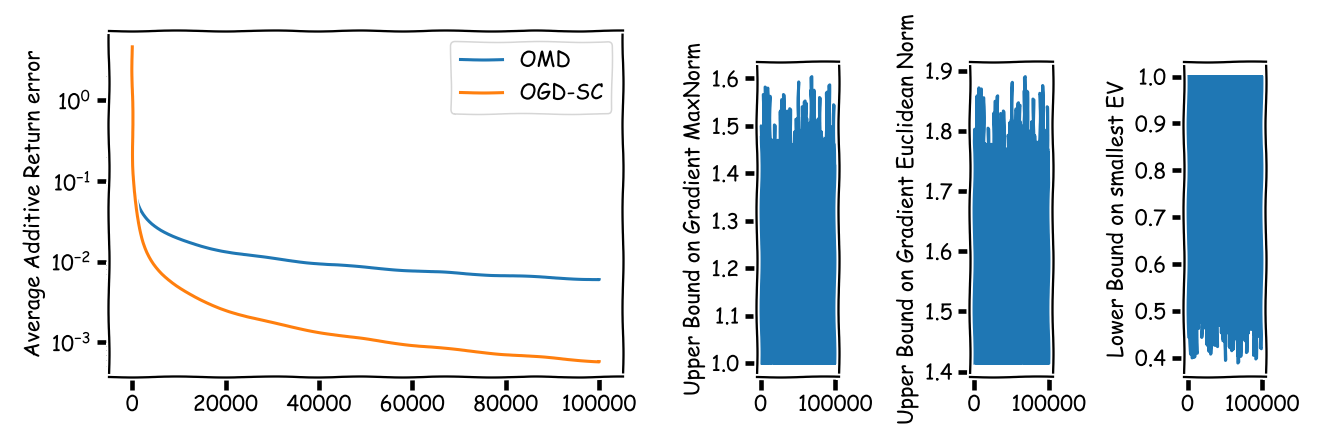This confirms our initial suspicion that (univPortOGDSC) is superior. Or is it? Let us consider another example but this time we take a more realistic number of $n = 50$ assets: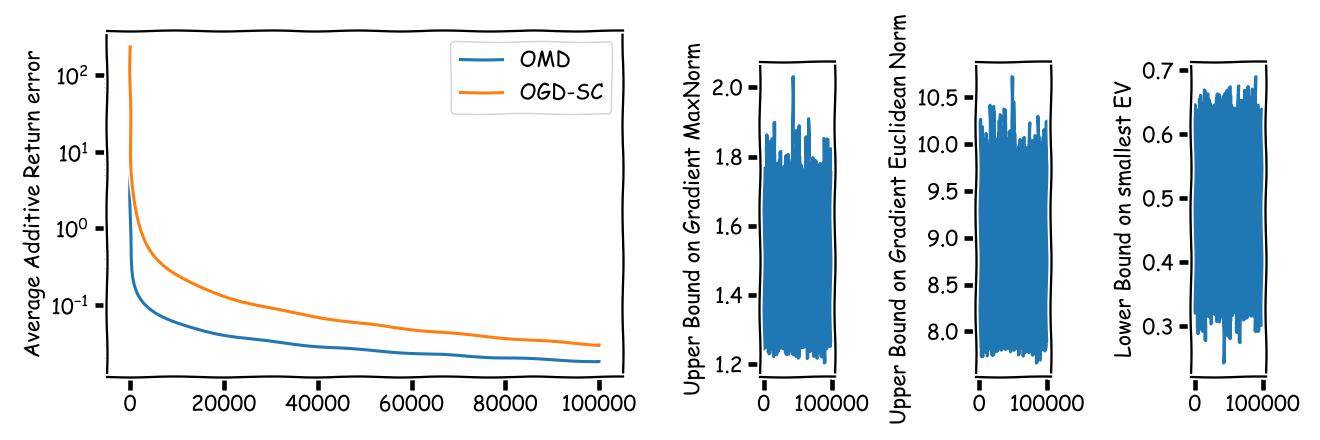This might be slightly surprising at first but what is happening is that (univPortOMD) scales much better due to the logarithmic dependence on the number of assets and the max norm of the gradients, whereas (univPortOGDSC) depends on the $2$-norm of the gradients and this scales roughly with $\sqrt{n}$ when the assets are reasonably independent. So basically OMD is slower in principle but starts from an exponentially lower initial error bound, so you need really long time horizons for (univPortOGDSC) to dominate (univPortOMD) for reasonably sized portfolios. In actual setups it really depends on the data and parameters in terms of which algorithm and bound performs better.

Moreover, as mentioned above what we really care for are the relative errors in the returns. For the same examples from above on the left we have the $2$-asset case and on the right the $50$-asset case.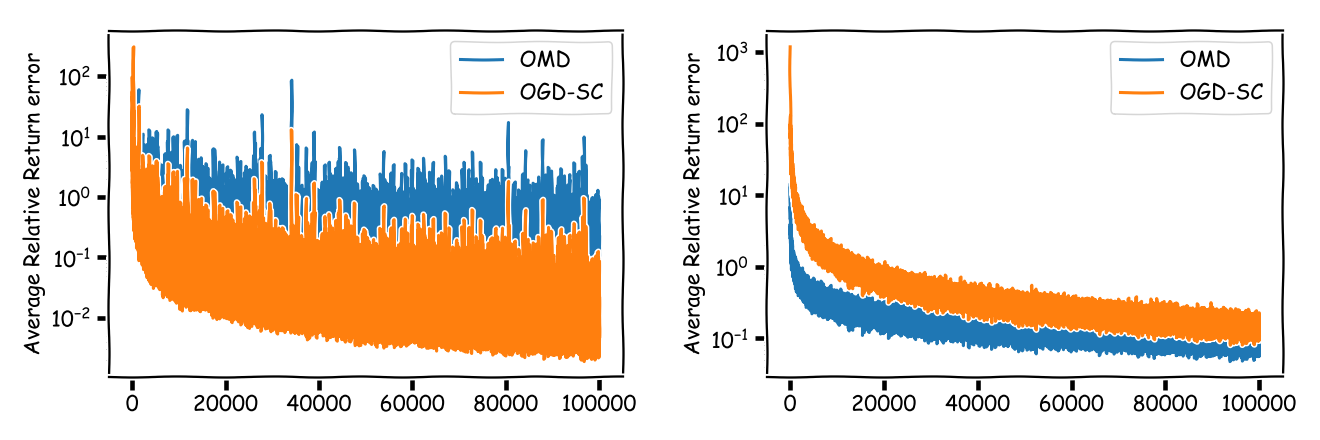Key takeaway is here that the relative error can easily be order an of magnitude worse than the additive error. In fact in both cases here, where we simulated really moderate markets, even after a large number of time steps the relative error is around $10\%$, which is still quite considerable.

### Actual time matters (more than anything)

The other important thing is that actual time not time steps matters a lot. This might be counterintuitive as well but bear with me. As can be seen from the two regret formulas of interest, (univPortOGDSC) and (univPortOMD), it is the number of time steps that is key to bringing down the average additive return error. As such, while we cannot accelerate time etc in the real-world, we might be tempted to trade more often. Let us see what happens. We consider a market that has $n = 50$ assets with a volatility of roughly that of the US equities market; the graph shows log (portfolio) value of the asset (as a single-asset portfolio).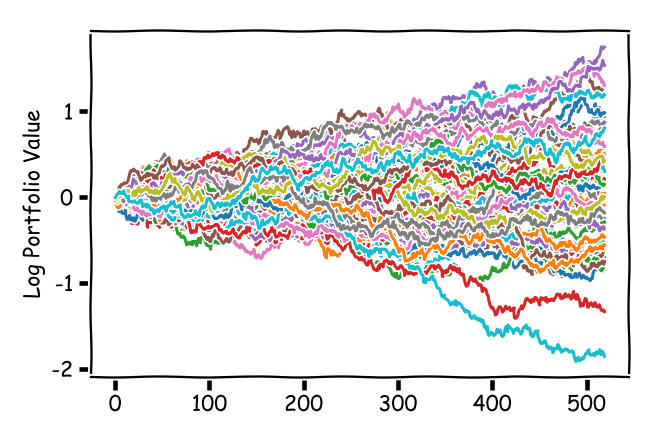Now in terms of trading and achievable average additive return errors in the next graphic, on the left we assume that we trade once per week, i.e., we have $T = 520$ time steps, whereas on the right we assume that we trade $100$ times per week, i.e., $T = 52000$.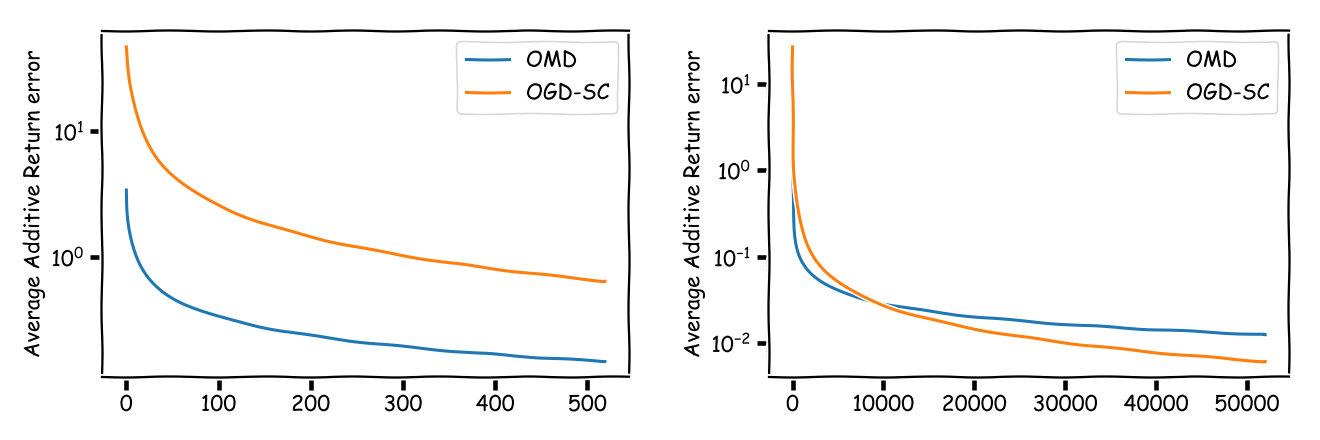First of all, we see that additive error for the once-per week setup is actually quite high, whereas on the right, trading $100$ times per week really helps to bring down the average additive error and we also see that (univPortOGDSC) starts to provide better guarantess than (univPortOMD). This is also consistent with many papers reporting tests over long(er) time horizons in order to ensure that the regret bounds are tight enough.

Now however, the story changes quite a bit when we consider the relative errors as shown in the next graphic.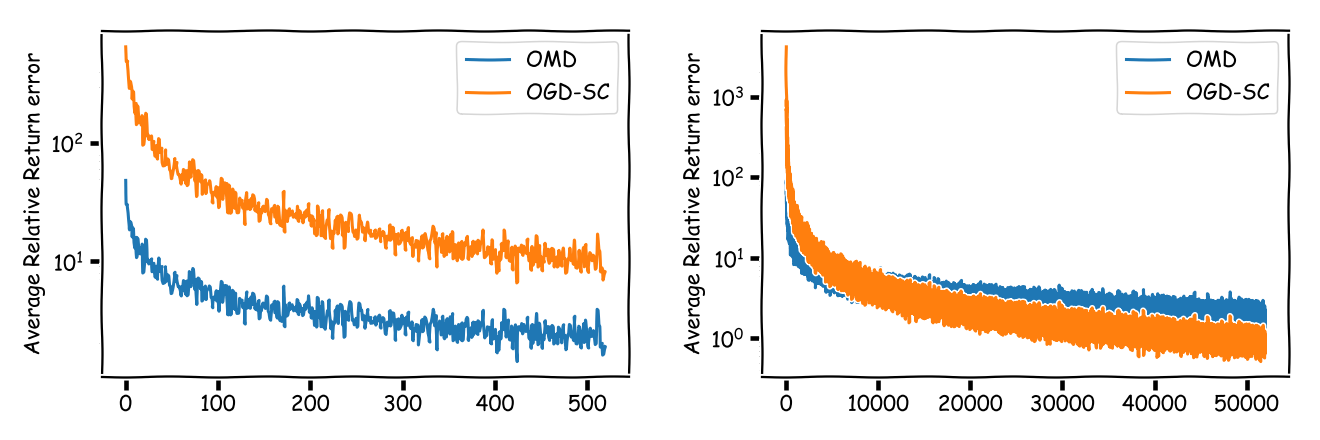So for (univPortOMD) basically nothing has changed. The reason is the following: what the increase in number of trades does is to basically turn $\sqrt{T}$ in the regret bound into $\sqrt{100 T} = \sqrt{100} \sqrt{T}$. However, the volatility of the underlying stock process scales the same way, i.e., the returns get roughly rescaled by $1/\sqrt{100}$ as well, so that (apart from a better tracking and removing discretization errors) we obtain basically the same relative errors. This is not the case for (univPortOGDSC) as it contracts quadratically faster (up to log factors), however the strong dependency on the number of assets might still outweigh this benefit.

In short: rescaling time by increasing the number of trades per time step is of limited efficiency. It is actual time that matters when we consider relative return errors, which is the quantity that actually matters.

Interlude: Understanding compounding or how to create a trust fund baby. Just to understand how important time is when it comes to investing and compounding, at retail investor return rates, say somewhere less than $8\%$ per year (which is already quite optimistic), within $20$ years of investing you can merely $4.7$-fold your initial investment; even $40$ years only give you a factor of about $22$, which is also not game changing: neither is going to make you rich when you started out poor and “getting rich” is closer to a factor $100$ increase in wealth). However, if you are willing not to invest for yourself but, say, for your grandchild, you have a good $60$ years of compounding and the situation is very different: you roughly $101.26$-folded your initial investment, say turning USD $10,000$ into roughly USD $1.00$ million. Go one generation further down the familty tree and it becomes USD $4.72$ million. So while it is unlikely that you can catapult yourself into the realm of riches (provided you did not start out rich), you do have the chance to do something for your family down the line. One might actually argue from an economic perspective the reason retail returns are not higher than they are is to maintain a certain equilibrium (while beyond the scope of this post: otherwise everybody would be rich which means nobody is rich). The lack of category-changing retail investment returns might also provide an explanation why people have been crazy about cryptocurrencies recently: they provide(d) a return that is potentially significant enough to gain $2$ generations of investing within a couple of years (or even months); at the correspondingly high risk levels though.

### Benchmarks on actual data

With the above in mind, let us benchmark (univPortOGDSC) and (univPortOMD), and hence the algorithms, in terms of achievable guarantees on actual market data.

#### SP500 stocks

In the first benchmark we consider the SP500 stocks from 01/01/2008 to 01/01/2018 in daily trading. After correcting for dropouts etc over the horizon we are left with $n = 426$ stocks and $T = 2519$ time steps (which corresponds to $10$ years of approx $252$ trading days/year). Apart from very basic tests, no further cleanup was done; that is left as an exercise to the reader. In the graphic below, on the left we have the actual market prices in USD of the assets and on the right the log (portfolio) value of the assets.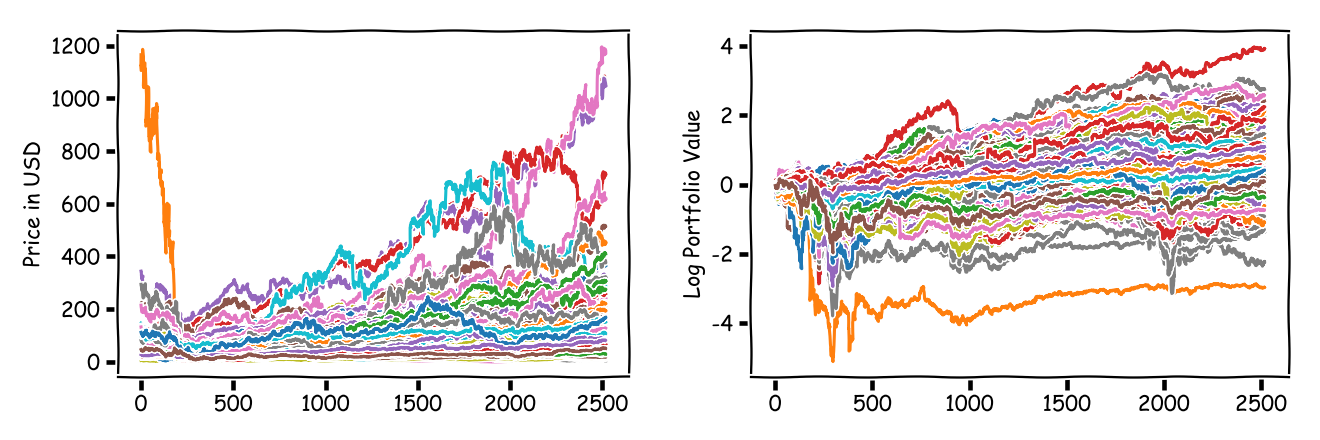In terms of achievable average additive return errors, they are huge for either of the bounds: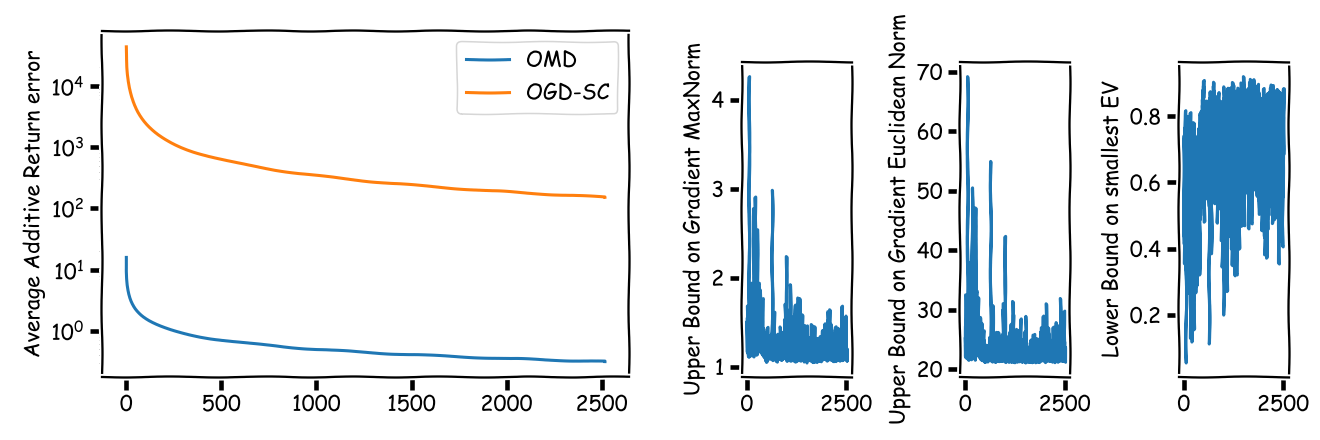We have roughly a “guarantee” of $100\%$ additive error in the daily return for the (better) OMD bound: to put this in perspective, say the best stock had a daily return of $3\%$ on average (that is huge), then we can “guarantee” an error band of $[-97\%,103\%]$ for the average daily performance of the universal portfolio at the end of the time horizon. Not quite helpful I guess. The situation gets much more pronounced in terms of average relative error: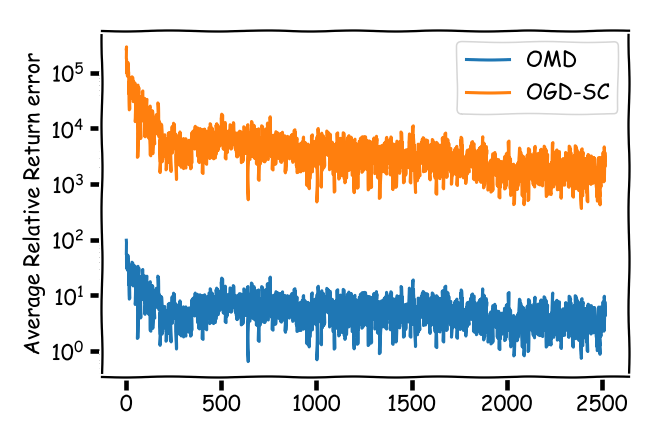#### Bitcoin

Maybe we were on the wrong end of the regime. Now let us consider fewer assets and a much higher trading frequency. We consider $n = 2$ assets: Bitcoin and a risk-free asset (e.g., cash or government bonds; unsure about the latter recently) that make up our portfolio. The risk-free asset returns a (moderate) $2\%$ over the full horizon. Those days you could consider yourself lucky to get any interest at all: Germany just tried to sell a zero-coupon bond at a crazy price (i.e., negative yield), which basically means parking your hard earned cash for $30$ years in a “safe-haven” and paying for it—demand at the auction was anemic. The original bitcoin market data was on a sub-second granularity level and for illustration here has been downsampled to $T = 566159$ time steps. As before, in the graphics below, on the left we have the actual market price in USD and on the right the log (portfolio) value of the two assets.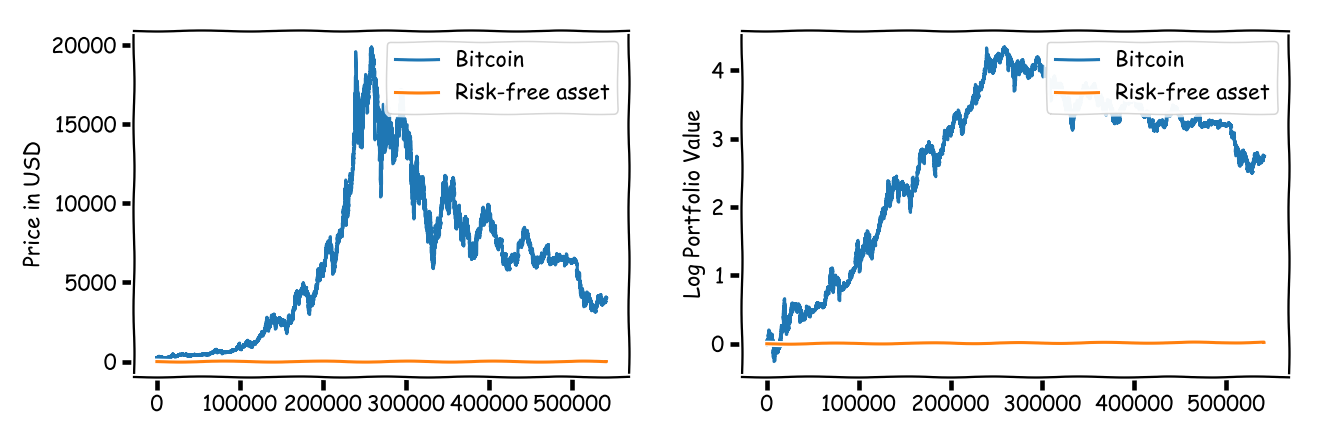Now, for our two regret bounds, we see that the variant utilizing the strong concavity performs much better (as we have only two assets, so that constants are small). In fact, $10^{-4}$ as average additive return error seems to be quite good.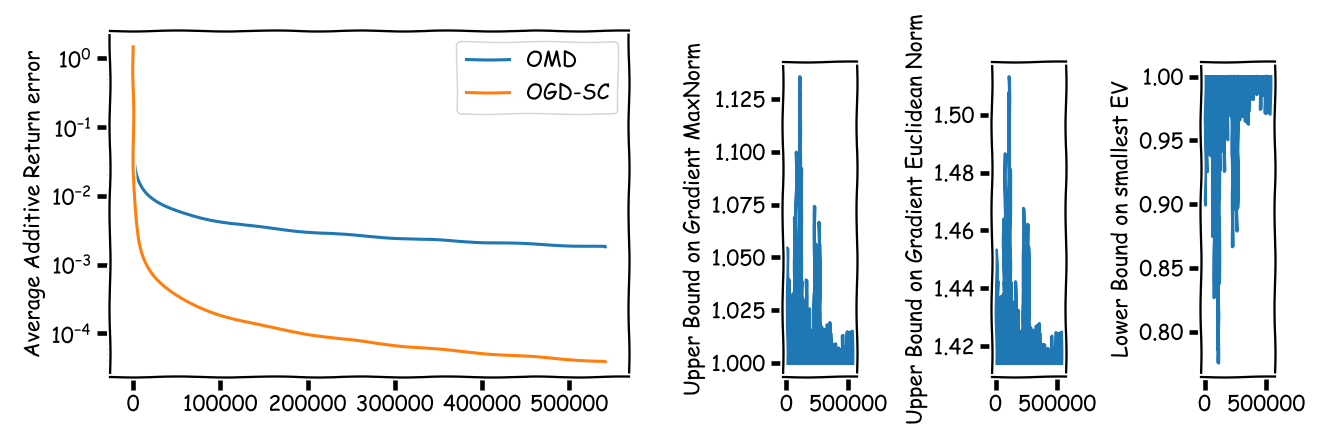However, unfortunately the reality here is a different one: average price changes between time steps are also quite small, so that the relative errors are huge: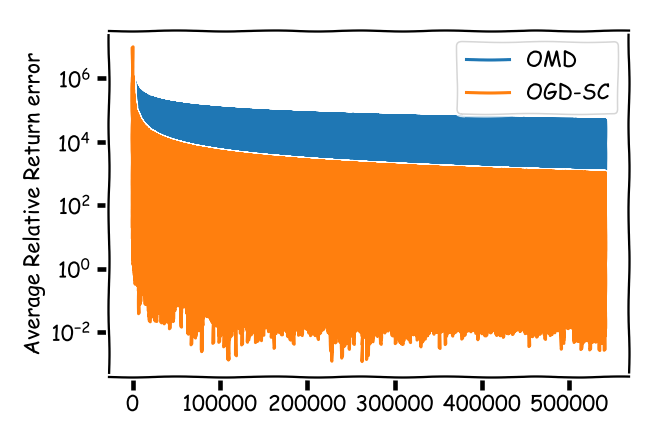Even towards the end of the time horizon our (guaranteed) average relative error bounds can be as large as $10^3$ making them impractical. Sure, here we have the special situation of only two assets, one of which is a risk-free asset so that the allocation cannot be that bad but that is beyond the point.

## A simple asymptotically optimal strategy

Although CRPs ensure competitiveness with respect to a wide variety of benchmarks (see above), in fact in most papers, the reference is often the single best asset in hindsight. This intuitively makes sense: you want to perform essentially (at least) as good as the best possible asset. Note that in principle CRPs can be even profitable when none of the assets is (as discussed before) but you need to basically have a situation where all assets have log geometric mean return of at most $0$. Once you are beyond this, i.e., there is an asset with strictly positive log geometric mean returns, the log portfolio value is optimized usually at a single asset.

### Split-and-Forget

So let us change our reference measure to not compete with the best CRP but with the best single asset in hindsight in view of this discussion. In this case there is a simple regret optimal strategy with constant regret in terms of terminal logarithmic wealth (as used in (univPortOGDSC) and (univPortOMD)). The strategy, let us call it Split-and-Forget, works as follows: Say we have $n$ assets, then simply allocate an $1/n$-fraction of capital to each of the $n$ assets and then forget about them (i.e., no rebalancing etc and hence also no additional transaction costs). Now let $V_t^i$ denote the value of asset $i$ at time $t$ and let $M_t$ denote the value of our portfolio at time $t$. Then the regret with respect to the best asset in hindsight is:

$\max_{i \in [n]} \log V^i_T - \log M_T \leq \max_{i \in [n]} \log V^i_T - \log \frac{1}{n} V^i_T = \log n,$

and as such, in the language from before, our average regret (i.e., average additive return error) satisifies

$\tag{SF} \max_{i \in [n]} \frac{1}{T }\log V^i_T - \frac{1}{T} \log M_T \leq \max_{i \in [n]}\frac{1}{T } \log V^i_T - \frac{1}{T} \log \frac{1}{n} V^i_T = \frac{\log n}{T},$

i.e., the bound has the logarithmic dependency on $n$ as OMD but an even higher (no log factor) convergence rate than OGD-SC. On top of that you have no transaction costs (except for the initial allocation). In fact, if the optimal CRP is attained by a single asset portfolio this basic strategy performs much better in terms of regret and achieved average returns. Note that [DGU]’s $1/n$-strategy rebalances to a uniform $1/n$ allocation at each rebalancing step and as such is different from Split-and-Forget, which does not alter the allocation after the split up in the initial step.

Interlude: Understanding diversification or why old families tend to be rich. In order to understand a bit better why the above is really working and actually not that bad of a strategy, observe that when doing the initial split up you pay for this once: In the worst-case the $\log n$ additive regret, which is the case where all but one asset is wiped out and you have only a $1/n$-fraction in the one remaining asset left. However, after the split up you are guaranteed that your portfolio is growing at a rate of the best single asset. More mathematically speaking, the split up leads to a shift by $\log n$ in the log portfolio value but after that its growth approaches optimal growth (compared to the single best asset). Sounds weird, but becomes more clear when you think of the asset $i$ compounding at some rate $r_i$. Now the terminal log portfolio wealth would be

$\log \sum_{i \in [n]} \frac{1}{n} e^{r_i T} = \log \sum_{i \in [n]} e^{r_i T - \log n},$

which is the LogSumExp (LSE) function (also sometimes called softmax), which for larger $T$ converges to the maximum: Let $j$ be so that $r_j$ is (uniquely) maximal, then

$\log \sum_{i \in [n]} e^{r_i T - \log n} = \log e^{r_j T - \log n} + \sum_{j \neq i \in [n]} \frac{e^{r_i T - \log n}}{e^{r_j T - \log n}} \rightarrow r_j T - \log n,$

so that the average return of the portfolio approaches $r_j - \frac{\log n}{T}$ as the fractions tend to $0$, which in turn approaches $r_j$ for larger $T$.

So what does this have to do with old families tending to be rich? Suppose at some point your family decided to split their wealth and invest equally into various business ventures, say $10$. You only need to get one of these ventures right and then, over time, you wash out your additive offset of $\log 10$; say the best one generates $4\%$ return per year, then it roughly takes $60$ years to “pay” for the cost of the split up. In other words, the cost of the split up translates into a shift in time and if you have enough time then you just wash out the cost. You might be well aware of the supercharged version of this: investing into startups. There you spread the capital even wider and try to harvest your black swan with explosive growth that pays for the losers in the portfolio and returns a hefty profit. This is simply convexity: the average of the individual payoffs can be much higher than the payoff of the average of the individuals.

### Split-and-Forget compared to OMD and OGD-SC

In the following we compare the realized average return errors (both additive and relative) of (SF) on the same examples that we have seen above. In each figure on the left we have the average additive error and on the right the average relative error.

The first one is the $50$ assets $10$ years example: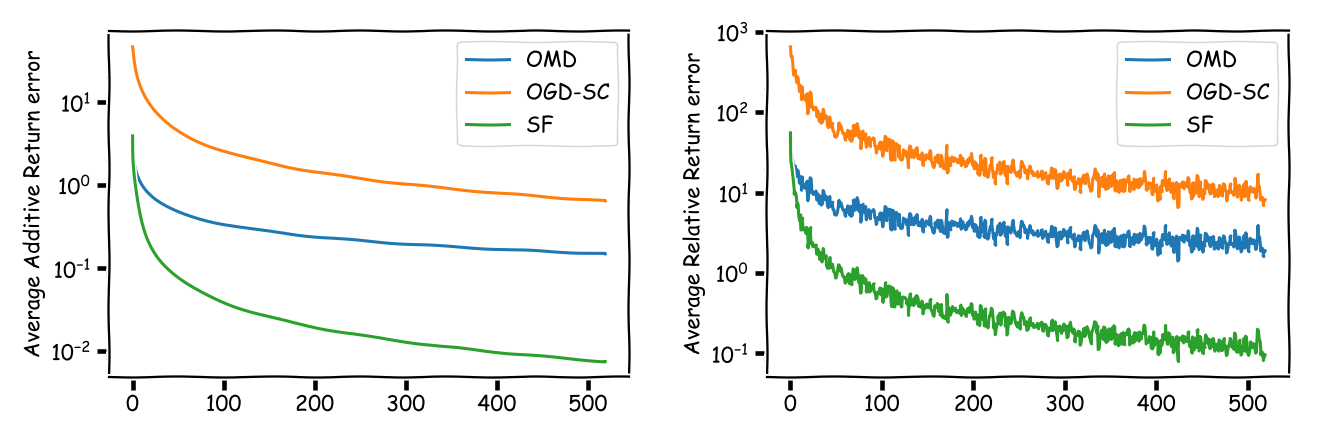And here the longer time horizon version used above to demonstrate the dependency on the constants: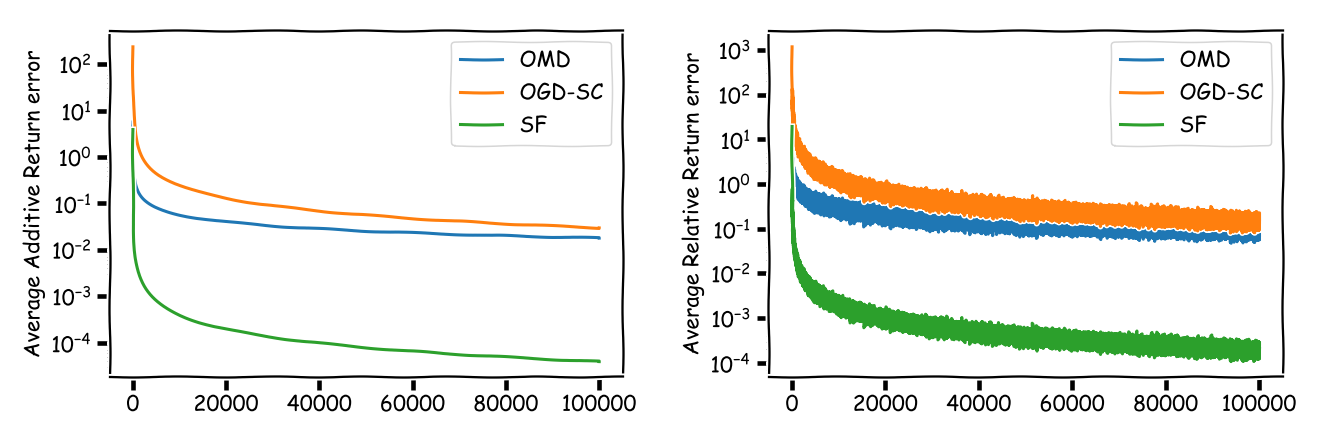The next one is the SP500 example from above: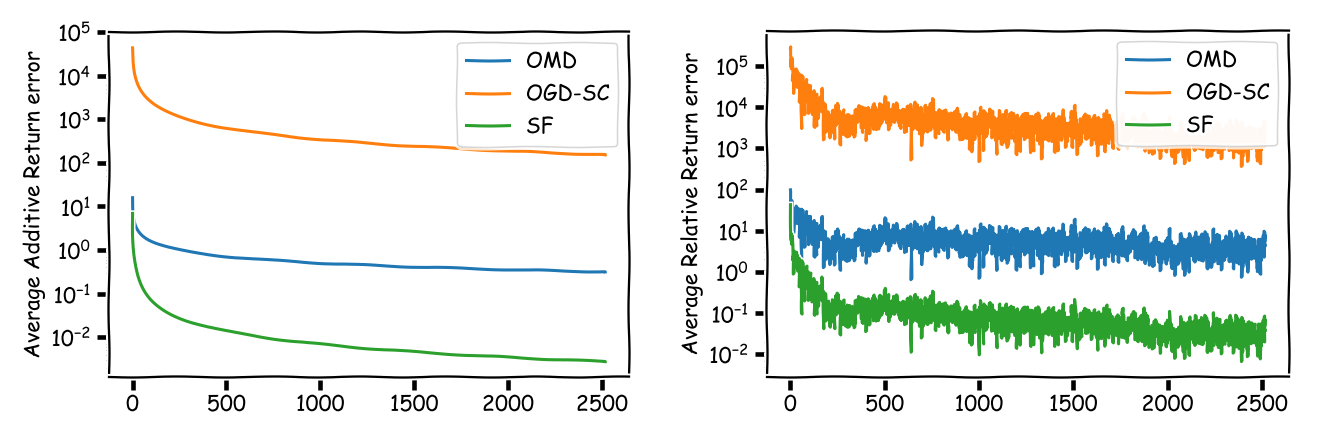And the bitcoin example: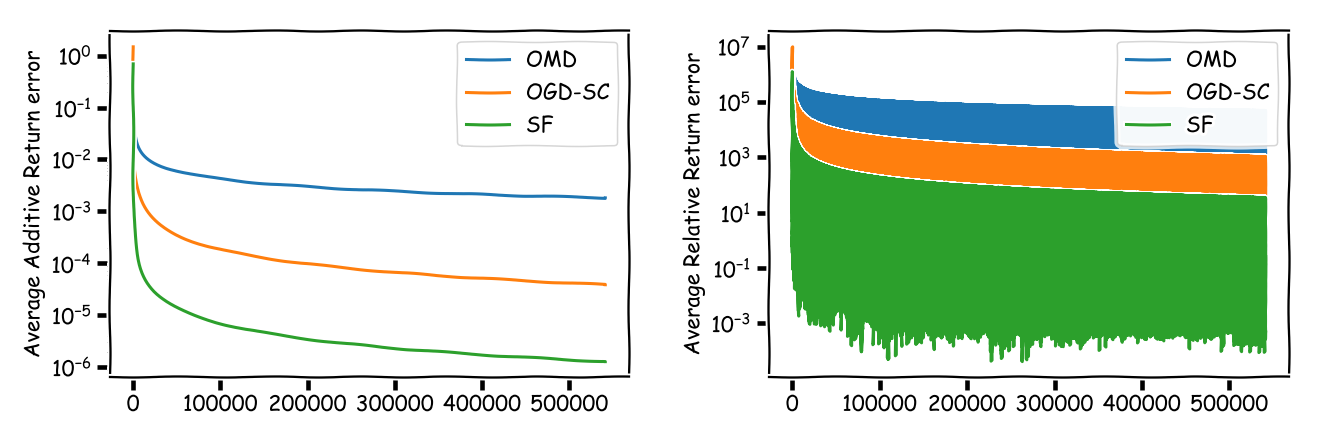As can be seen the regret of (SF) is significantly smaller than the regret of (OGD-SC) and (OMD) albeit with respect to a slightly different benchmark: the best single asset vs. the best CRP. We can have now a very long discussion about whether (SF) is a good strategy or not. What the (SF) strategy however does is, it sheds some light on what one can really expect from universal portfolios.

In actual backtesting performance the algorithms (as usual) perform much better, although I want to stress this is without guarantee and hence puts us back into the realms of justification-by-backtesting; you should always validate by backtesting though. Thus, just for completeness a quick backtesting experiment. Here we ran the actual algorithms (as compared to just computing the additive and relative bounds) and benchmark the (SF) strategy against the, for this case better suited, (OGD-SC) strategy. The green curve “Opt” is the in-hindsight-optimal CRP allowing for leverage of a factor of up to $2$, i.e., it is outside of the benchmarking class of CRPs and I added it just for comparison. You can clearly see the offset of (SF) at the start and see that this offset translates into a time shift from where onwards it dominates (OGD-SC) significantly in growth rate, essentially following the market: negative offset but higher rate.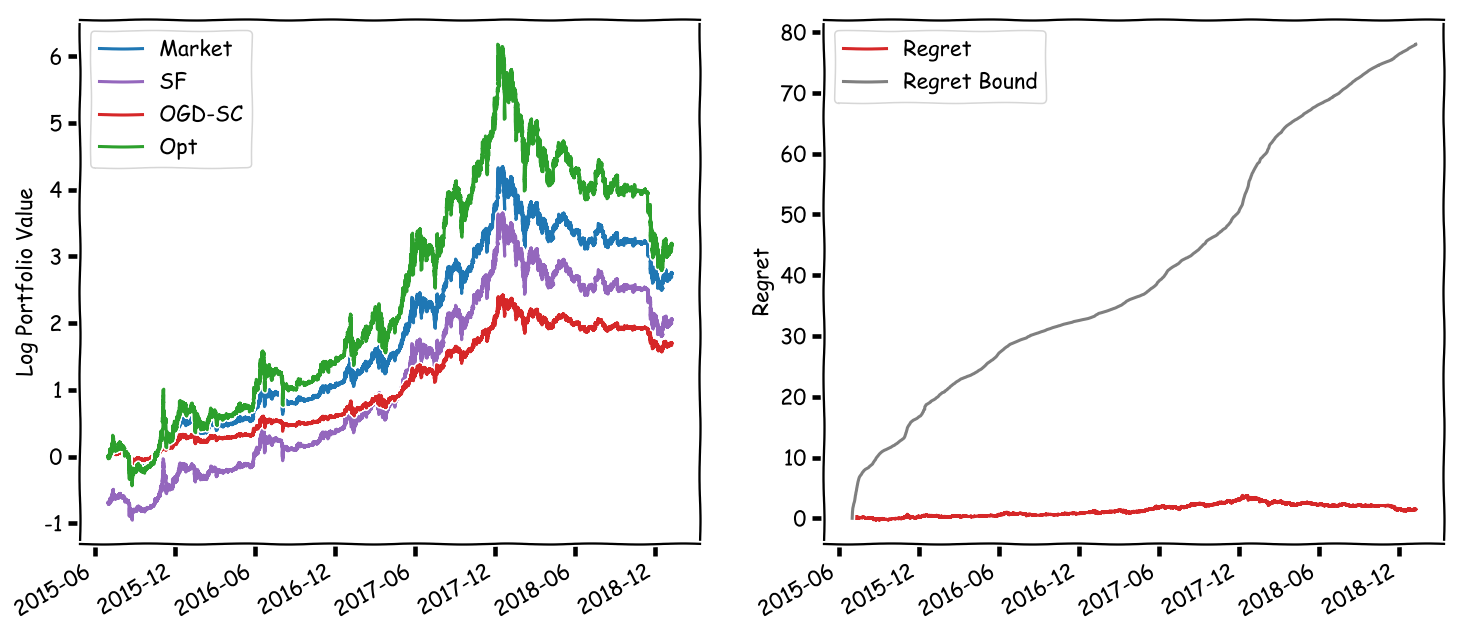[K] Kelly Jr, J. L. (2011). A new interpretation of information rate. In The Kelly Capital Growth Investment Criterion: Theory and Practice (pp. 25-34). (original article from 1956) pdf

[L] Latane, H. A. (1959). Criteria for choice among risky ventures. Journal of Political Economy, 67(2), 144-155. pdf

[S] Shannon, C. E. (1948). A mathematical theory of communication. Bell system technical journal, 27(3), 379-423. pdf

[C] Cover, T. M. “Shannon and investment.” IEEE Information Theory Society Newsletter, Summer (1998). pdf

[T] Thorp, E. O. (1966). Beat the Dealer: a winning strategy for the game of twenty one (Vol. 310). Vintage.

[TK] Thorp, E. O., & Kassouf, S. T. (1967). Beat the market: a scientific stock market system. Random House.

[B] Breiman, L. (1961). Optimal gambling systems for favorable games. pdf

[P] Poundstone, W. (2010). Fortune’s formula: The untold story of the scientific betting system that beat the casinos and Wall Street. Hill and Wang.

[MTZZ] MacLean, L. C., Thorp, E. O., Zhao, Y., & Ziemba, W. T. (2011). How Does the Fortune’s Formula Kelly CapitalGrowth Model Perform?. The Journal of Portfolio Management, 37(4), 96-111. pdf

[T2] Thorp, E. O. (2011). Understanding the Kelly criterion. In The Kelly Capital Growth Investment Criterion: Theory and Practice (pp. 509-523).

[T3] Thorp, E. O. (2011). The Kelly criterion in blackjack sports betting, and the stock market. In The Kelly Capital Growth Investment Criterion: Theory and Practice (pp. 789-832).pdf

[C1] Cover, T. (1984). An algorithm for maximizing expected log investment return. IEEE Transactions on Information Theory, 30(2), 369-373. pdf

[C2] Cover, T. M. (2011). Universal portfolios. In The Kelly Capital Growth Investment Criterion: Theory and Practice (pp. 181-209). (original reference: Cover, T. M. (1991). Universal Portfolios. Mathematical Finance, 1(1), 1-29.) pdf

[CO] Cover, T. M., & Ordentlich, E. (1996). Universal portfolios with side information. IEEE Transactions on Information Theory, 42(2), 348-363. pdf

[BK] Blum, A., & Kalai, A. (1999). Universal portfolios with and without transaction costs. Machine Learning, 35(3), 193-205. pdf

[KV] Kalai, A., & Vempala, S. (2002). Efficient algorithms for universal portfolios. Journal of Machine Learning Research, 3(Nov), 423-440. pdf

[HSSW] Helmbold, D. P., Schapire, R. E., Singer, Y., & Warmuth, M. K. (1998). On‐Line Portfolio Selection Using Multiplicative Updates. Mathematical Finance, 8(4), 325-347. pdf

[Z] Zinkevich, M. (2003). Online convex programming and generalized infinitesimal gradient ascent. In Proceedings of the 20th International Conference on Machine Learning (ICML-03) (pp. 928-936). pdf

[NY] Nemirovsky, A. S., & Yudin, D. B. (1983). Problem complexity and method efficiency in optimization.

[AHK] Arora, S., Hazan, E., & Kale, S. (2012). The multiplicative weights update method: a meta-algorithm and applications. Theory of Computing, 8(1), 121-164. pdf

[HAK] Hazan, E., Agarwal, A., & Kale, S. (2007). Logarithmic regret algorithms for online convex optimization. Machine Learning, 69(2-3), 169-192. pdf

[DGU] DeMiguel, V., Garlappi, L., & Uppal, R. (2007). Optimal versus naive diversification: How inefficient is the 1/N portfolio strategy?. The review of Financial studies, 22(5), 1915-1953. pdf

#### Changelog

09/07/2019: Fixed several typos and added reference [DGU] as pointed out by Steve Wright.